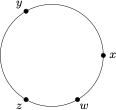# 每日一题循环阵列

A．$\left( {y,z,w} \right) \in S$，$\left( {x,y,w} \right) \notin S$

B．$\left( {y,z,w} \right) \in S$，$\left( {x,y,w} \right) \in S$

C．$\left( {y,z,w} \right) \notin S$，$\left( {x,y,w} \right) \in S$

D．$\left( {y,z,w} \right) \notin S$，$\left( {x,y,w} \right) \notin S$